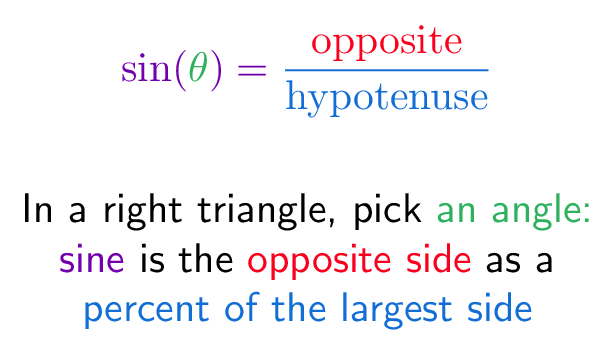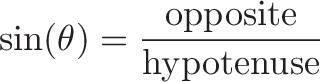# Sine (Geometric Definition)

## Colorized Definition\newcommand{\sinefunction}{\color{c1}}
\newcommand{\thetacolor}{\color{c2}}
\newcommand{\opposite}{\color{c3}}
\newcommand{\hypotenuse}{\color{c4}}$\displaystyle{ \sinefunction \sin(\thetacolor{\theta} \sinefunction) = \hypotenuse \frac{\opposite \textrm{opposite}}{\textrm{hypotenuse}} }$

\plain In a right triangle, pick \thetacolor an angle:
\
\sinefunction sine \plain is the \opposite opposite side
\plain as a
\
\hypotenuse percent of the largest side

## Read More - [How to learn Trigonometry Intuitively](https://betterexplained.com/articles/intuitive-trigonometry/) - [Intuitive Understanding of Sine Waves](https://betterexplained.com/articles/intuitive-understanding-of-sine-waves/)